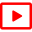## Week 4week 4 overview

• 7. Discrete symmetries of dynamics
• What is a symmetry of laws of motion? The families of symmetry-related full state space cycles are replaced by fewer and often much shorter "relative" cycles, and the notion of a prime periodic orbit is replaced by the notion of a "relative" periodic orbit, the shortest segment that tiles the cycle under the action of the group. Discrete symmetries: a review of the theory of finite groups.Chapter 10 Flips, slides and turnsDiscrete symmetries: 3-disk pinballWhat is a group?Active, passive coordinate transformationsFollowing Mefisto: symmetry defined three (3) timesExample: a 1-dimensional system with a symmetrySubgroups, classes, group orbitsY. Feng, part 2: From matrix reps to fundamental domain complete PP: Symmetry and Group Theory

• 8. Discrete symmetry reduction of dynamics to a fundamental domain
• While everyone can visualize the fundamental domain for a 3-disk billiard, the simpler problem - symmetry reduction of 1d dynamics that is equivariant under a reflection, the most common symmetry in applications - seems to baffle everyone. So here is a step-by-step walk through to this simplest of all symmetry reductions.Chapter 11 World in a mirrorChapter 11 slidesFundamental domainD. Lippolis : Discrete symmetry reduction - examples (60 min)D. Lippolis : slides

• Homework 4
•discrete symmetry reductionDiscussion forum for week 5

• Optional
•Handwritten: a 1-dimensional fundamental domain "It does not say anyplace in the Bible that if equations of motion have a symmetry, solutions should have it too."